| Technical Writer/Review: ABCOM Team | Level: Intermediate | Banner Image Source : Internet |

# Motivation

Last few years, we have seen the rapid penetration of Machine Learning in the BFSI (Banking, Financial Services and Insurance) sector. To cite a few examples, the COiN (Contract Intelligence) platform by JPMorgan Chase and AI Chatbots by Wells Fargo and Privatbank may be noted. So what keeps the Insurance sector away from AI/ML? No, this is not really true. Insurance industry wants ML and there are many successful stories. However, there are several challenges faced while developing ML models in this industry. Some of the key factors that influence the decision-making process may be listed below:

• Extremely complex underwriting rule-sets
• The rule-sets radically differ across the product lines
• Many non-KYC (Know Your Customers) cases
• Lack of centralized information base
• Inertia in regulatory compliance

Having considered the challenges, I will now provide you with a case study for risk underwriting in Health Insurance. I will show you the effect of various attributes of the insured and how they would affect his insurance premium.

# ML Model Choice

It is well known that the Gradient Boosting algorithm is one of the most powerful machine learning techniques for building predictive models for both classification and regression problems.

• A loss function to be optimized - There are several loss functions, and the choice depends on the problem that you are solving.
• A weak learner - Decision trees are the learners in gradient boosting. We construct greedy trees to minimize the loss.
• Trees are added one at a time and existing trees in the model are not altered. A gradient descent style is used to minimize the loss while adding trees.

At the end, we use the weak learner for production and predicting unknown data points.

A few important advantages of the algorithm are:

• Provides a far superior predictive accuracy compared to others
• Lots of flexibility - can optimize on different loss functions and provide several hyper-parameter tuning options that provide lots of flexibility in model fitting.
• No data pre-processing required - often works great with categorical and numerical values as is.

Now, I will give you some reasoning for using the Gradient Boosting algorithm for this project. The dataset used in this project contains a categorical feature that would make you immediately jump to the selection of a tree-based algorithm. Second, selection of regression or SVM-based regression would require further data processing like scaling and normalization.

Having said all this, now let us start with understanding the problem statement.

# Application

I took the dataset that I am going to use for this project from Kaggle. It comprises the following fields:

• Age: age of the beneficiary
• Sex: Gender of the beneficiary
• BMI: The Body mass index of the beneficiary
• Children: Number of children covered by health insurance / Number of dependents.
• Smoker: Does the beneficiary smoke or not.
• Region: the beneficiary’s residential area
• Insurance Charges: The insurance costs or the billed individual medical costs

Our task is to develop an efficient ML model that will help the insurance company in deciding on the insurane premium, given the attributes for a new customer.

Let us begin with the project creation.

# Project

Create a new Colab project and rename it to InsuranceClaim.

Import the following packages:

``````import numpy as np
import pandas as pd
import matplotlib.pyplot as plt
import warnings
import seaborn as sns
from sklearn.metrics import accuracy_score,r2_score,mean_absolute_error,mean_squared_error
from sklearn.model_selection import train_test_split
from sklearn.preprocessing import LabelEncoder
warnings.filterwarnings("ignore")
``````

We will be using the `GradientBoostingRegressor` from sklearn.

I have uploaded the dataset on our GitHub for your quick access in the Colab project. Load the data into your project using the following command:

``````df=pd.read_csv("https://raw.githubusercontent.com/abcom-mltutorials/Datasets_general/main/insurance.csv"
``````

Examine few records of the loaded data:

``````df.head()
``````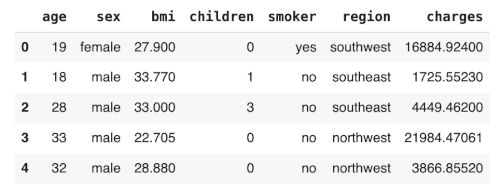## Data Cleaning

First, check if the data has any null values.

``````df.isnull().sum()
``````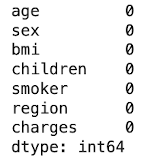From the output, we can observe that our dataset doesn’t have any missing values in it. Now, let us preprocess the data.

## Data Preprocessing

Let us first examine the dataset structure:

``````df.info()
``````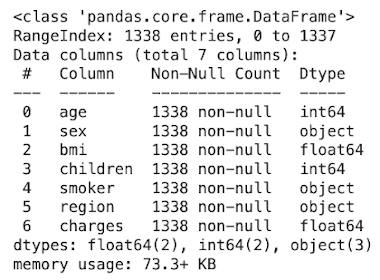We observe the features sex, smoker, and region are of object data type. We will need to encode these fields by using either the label-encoding or one-hot encoding. Before performing any transformation, understand these categorical data points. We will use `pandas.DataFrame.unique` function to return unique values available in these features.

``````print("Unique classes in Sex : ",df['sex'].unique())
print("Unique classes in smoker : ",df['smoker'].unique())
print("Unique classes in region : ",df['region'].unique())
``````

This is the output:

``````Unique classes in Sex :  ['female' 'male']
Unique classes in smoker :  ['yes' 'no']
Unique classes in region :  ['southwest' 'southeast' 'northwest' 'northeast']
``````

We will use the `LabelEncoder` to transform these labels. If the number of classes is large, we would prefer using one-hot encoding.

``````labelencoder = LabelEncoder()
df['sex']=labelencoder.fit_transform(df['sex'])
df['smoker']=labelencoder.fit_transform(df['smoker'])
df['region']=labelencoder.fit_transform(df['region'])
``````

After encoding, let us examine the data one more time: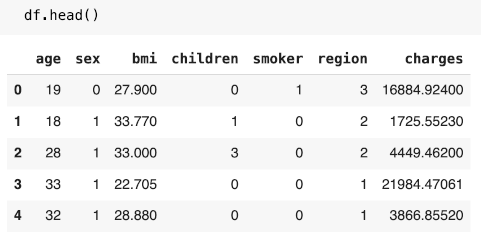The output shows that all our categorical variables are converted into numerical values. Now, let us do some EDA (Exploratory Data Analysis) to understand the data.

## Exploring Data

We will do a scatter plot for age against the charges with respect to the smoker feature.

``````plt.figure(figsize=(8,6))
sns.scatterplot(df.age,df.charges,hue=df.smoker,palette= ['green','red'] ,alpha=0.6)
plt.show()
``````

This is the output: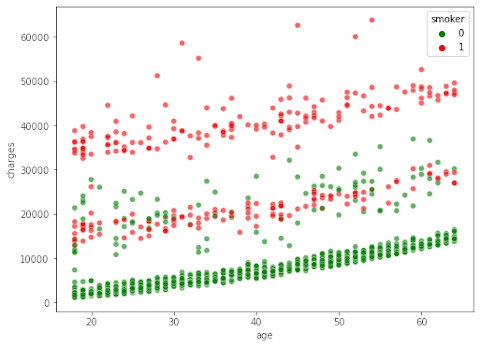From the output, we understand that the charges for premium claims increase with age and also increase substantially for smokers. We can derive that age and smoker are crucial features for training the model.

## Features/Target

As said earlier, our target for model training is the charges column. We will use the rest of the fields as the features.

We extract the features using the following statement:

``````X=df.drop("charges",axis=1)
``````

We create the target variable using indexing :

``````y=df.charges
``````

## Training/Testing Datasets

We split the dataset into training and testing using the following statement:

``````x_train,x_test,y_train,y_test=train_test_split(X,y,test_size=0.2,random_state=123)
print("train: ",x_train.shape)
print("test: ",x_test.shape)
``````

Output:

``````train:  (1070, 6)
test:  (268, 6)
``````

We reserved 20% of data for testing. From the output, we know that the training dataset consists of 1070 data points.

At this point, we are done with data preparation, so let us proceed to model building.

# Model

As we mentioned earlier, we will use the Gradient Boosting algorithm to perform the regression task. Sci-kit learn has the implementation of the Gradient Boosting Algorithm under its ensemble API. One advantage of using this algorithm is that it does not require scaling or normalization of features, unlike other algorithms like KNN, SVM, or linear regression.

``````model=GradientBoostingRegressor(n_estimators=433,
loss='huber',
learning_rate=0.056767559947689034,
criterion='mse',
max_depth=3,
max_features='auto',
tol=0.001,
n_iter_no_change=10,
verbose=1,
random_state=123)
``````

We use huber as the loss function. Here is the brief description of the rest of the parameters:

• N_estimators: The number of boosting stages to perform. Gradient boosting is fairly robust to over-fitting so a large number usually results in better performance.
• Loss: Loss function to be optimized.
• Learning_rate: Learning rate shrinks the contribution of each tree by learning_rate. There is a trade-off between learning_rate and n_estimators.
• Criterion: The function to measure the quality of a split.
• Max_depth: Maximum depth of the individual regression estimators.
• Max_features: The number of features to consider when looking for the best split
• Tol: Tolerance for the early stopping. When the loss is not improving by at least tol for n_iter_no_change iterations

You would wonder from where did I decide on those parameter values in the above statement? Rather than using some default values, I used the fine-tuned parameters generated by Optuna. I will describe Optuna and how it is used for getting best-fitting parameters in another upcoming tutorial.

After we initialize the model, we are ready to perform the actual training operation on the model by calling the `fit` function along with the training sets as its arguments.

``````model.fit(x_train,y_train)
``````

After the model is trained, we evaluate its performance.

## Model Evaluation

We estimate the generalization accuracy of a model on unseen data by checking the model’s accuracy score.

``````print("accuracy test:{:.2f}%".format(model.score(x_test,y_test)*100))
``````

Output:

``````accuracy test: 91.09%
``````

Another way of evaluation is by making a prediction on the test dataset and comparing the actual test values to the predicted values.

``````y_pred=model.predict(x_test)
print("r2_score on test set : {:.2f} %".format(r2_score(y_test,y_pred)))
``````

Output:

``````r2_score on test set : 0.91 %
``````

In the above code snippet, we used R2 score to evaluate the prediction of our model. Where `R2` or `R-squared` is a goodness-of-fit measure for regression models. These statistics show the percentage of the variance in the dependent variable that the independent variables explain collectively.

## Feature Importance

Feature importance refers to a class of procedures for allocating scores to input features to a predictive model that shows the relative importance of each feature when making a prediction.

Decision Tree-based Algorithms can calculate feature importance while training a model. By using the f`eature_importances_ attribute` of `GradientBoostingRegressor`, we can plot the feature importance plot for the data.

``````plt.figure(figsize=(8,6))
n_features = len(X.columns)
plt.barh(range(n_features),model.feature_importances_, align='center')
plt.yticks(np.arange(n_features), X.columns)
plt.xlabel("Feature importance")
plt.ylabel("Feature")
plt.ylim(-1, n_features)
``````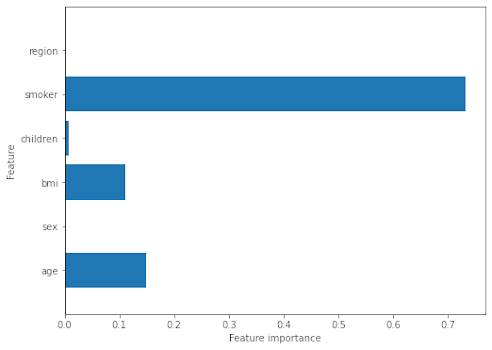From the plot, we see that the feature smoker has the highest impact on prediction, followed by age and BMI. The sex and region have the least impact.

Now, let us try to use the model on an unseen data.

## Inference on Unseen

We will now create a test data point and observe how the model infers on it:

Consider a customer is an 18-year-old male and has a BMI of 25.002. He doesn’t smoke and stays in the northeast region. Let us see what amount our model predicts for this test case and let us also check what our model would predict if the same beneficiary was a smoker:

We will add the following data into a dataframe for testing.

index Age sex bmi Children Smoker Region
0 18 1 25.002 0 0 0
1 18 1 25.002 1 0 0
``````data={'age':[18,18],'sex':[1,1],'bmi':[25.002,25.002],'children':[0,0],'smoker':[0,1],'region':[0,0]}
my_test_data=pd.DataFrame(data=data)
my_test_data
``````

Output: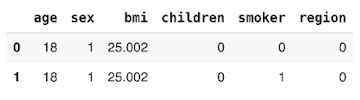Now let's make prediction on this test case and observe the impact of features

``````result=model.predict(my_test_data)
print("output : ",result)
``````

Output:

``````output :  [ 5566.69343641 19936.14511967]
``````

We observe that the impact on charges changes from 5566 for a non-smoker to 19936 for a smoker with all other attributes unchanged.

# Conclusion

In the tutorial, you learned how to develop a Machine learning model using the Gradient Boosting algorithm applied in the insurance sector. It is important for the insurance company to know which features impact the premium most. You learned how to study the impact of the various features on the target value.